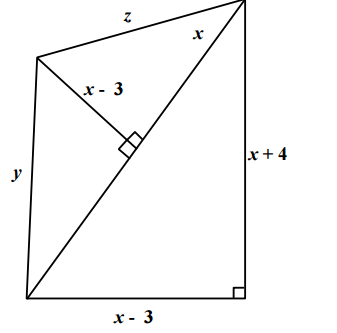Question 42

# Based on the figure below, what is the value of x, if y = 10?Solution

We will solve this question by taking the options.

Suppose x = 11

x+4 = 15, x-3 = 8

Hyptenuse = $$\sqrt{225+64} = 17$$

17-11 = 6

$$6^2+(x-3)^2 = y^2$$

x-3 = 8

y = 10 which is similar to what is given in the question.

Hence x = 11

• All Quant CAT Formulas and shortcuts PDF
• 30+ CAT previous papers with solutions PDF